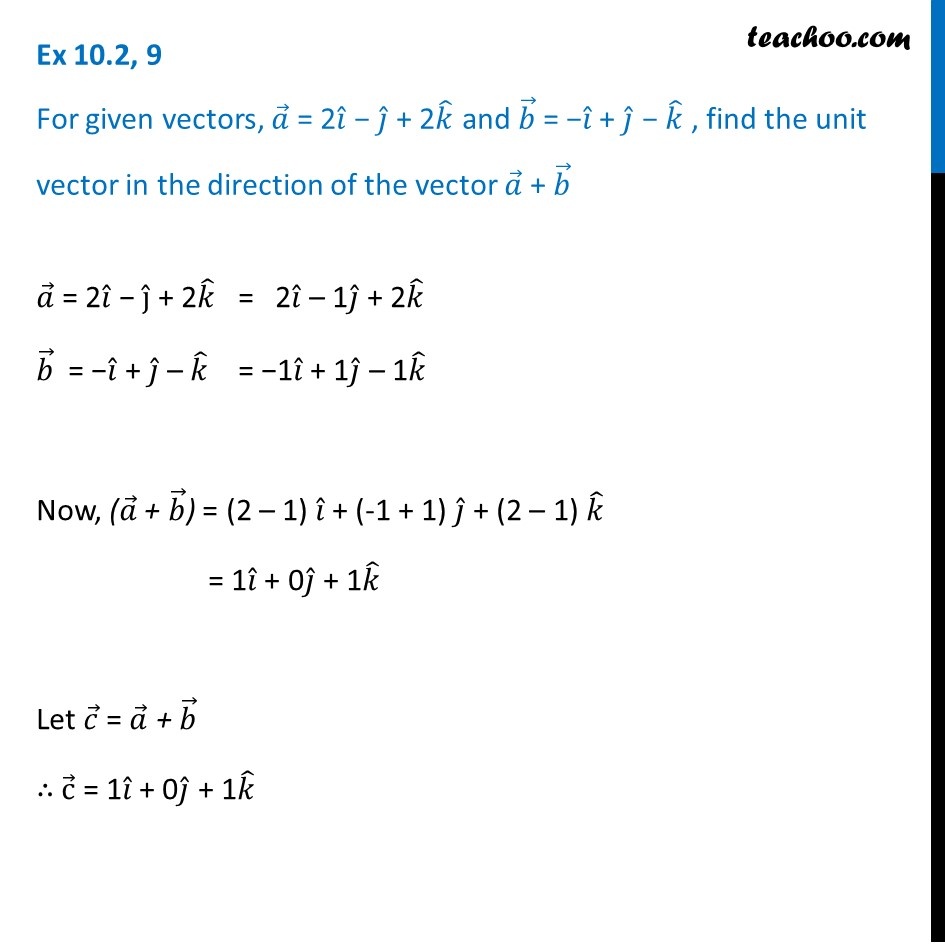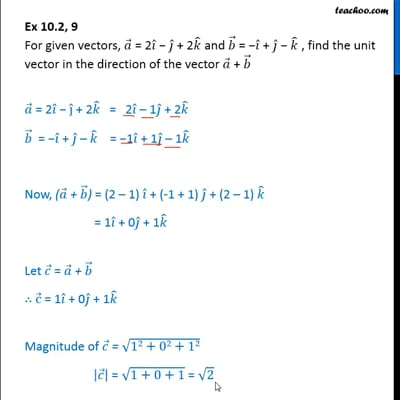Ex 10.2

Chapter 10 Class 12 Vector Algebra
Serial order wiseThis video is only available for Teachoo black users

Solve all your doubts with Teachoo Black (new monthly pack available now!)

### Transcript

Ex 10.2, 9 For given vectors, 𝑎 ⃗ = 2𝑖 ̂ − 𝑗 ̂ + 2𝑘 ̂ and 𝑏 ⃗ = −𝑖 ̂ + 𝑗 ̂ − 𝑘 ̂ , find the unit vector in the direction of the vector 𝑎 ⃗ + 𝑏 ⃗𝑎 ⃗ = 2𝑖 ̂ − j ̂ + 2𝑘 ̂ = 2𝑖 ̂ – 1𝑗 ̂ + 2𝑘 ̂ 𝑏 ⃗ = −𝑖 ̂ + 𝑗 ̂ – 𝑘 ̂ = −1𝑖 ̂ + 1𝑗 ̂ – 1𝑘 ̂ Now, (𝑎 ⃗ + 𝑏 ⃗) = (2 – 1) 𝑖 ̂ + (-1 + 1) 𝑗 ̂ + (2 – 1) 𝑘 ̂ = 1𝑖 ̂ + 0𝑗 ̂ + 1𝑘 ̂ Let 𝑐 ⃗ = 𝑎 ⃗ + 𝑏 ⃗ ∴ c ⃗ = 1𝑖 ̂ + 0𝑗 ̂ + 1𝑘 ̂ Magnitude of 𝑐 ⃗ = √(12+02+12) |𝑐 ⃗ | = √(1+0+1) = √2 Unit vector in direction of 𝑐 ⃗ = 1/|𝑐 ⃗ | . 𝑐 ⃗ 𝑐 ̂ = 1/√2 [1𝑖 ̂+0𝑗 ̂+1𝑘 ̂ ] 𝑐 ̂ = 1/√2 𝑖 ̂ + 0𝑗 ̂ + 1/√2 𝑘 ̂ 𝑐 ̂ = 𝟏/√𝟐 𝒊 ̂ + 𝟏/√𝟐 𝒌 ̂ Thus, unit vector in direction of 𝑐 ⃗ = 1/√2 𝑖 ̂ + 1/√2 𝑘 ̂